# High School Math : How to find the surface area of a sphere

## Example Questions

2 Next →

### Example Question #151 : Solid Geometry

What is the surface area of a sphere with a radius of?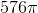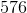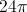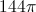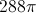Explanation:

To solve for the surface area of a sphere you must remember the formula: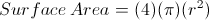First, plug the radius into the equation for: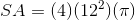Since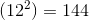, the surface area is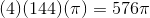.

The answer is therefore.

2 Next →